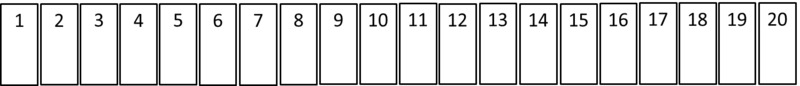# The Locker Game

Alignments to Content Standards: 4.OA.B.4

The 20 students in Mr. Wolf's 4th grade class are playing a game in a hallway that is lined with 20 lockers in a row.• The first student starts with the first locker and goes down the hallway and opens all the lockers.
• The second student starts with the second locker and goes down the hallway and shuts every other locker.
• The third student stops at every third locker and opens the locker if it is closed or closes the locker if it is open.
• The fourth student stops at every fourth locker and opens the locker if it is closed or closes the locker if it is open.

This process continues until all 20 students in the class have passed through the hallway.

1. Which lockers are still open at the end of the game? Explain your reasoning.
2. Which lockers were touched by only two students? Explain your reasoning.
3. Which lockers were touched by only three students? Explain your reasoning.
4. Which lockers were touched the most?

## IM Commentary

The purpose of this instructional task is for students to deepen their understanding of factors and multiples of whole numbers. This is a classic mathematical puzzle; often it is stated in terms of 100 lockers, so students have to make the connection to factors and multiples to solve it. In this version, students can just go through all the rounds of opening and closing locker doors and observe after the fact that there is a relationship between the factors a locker number has and whether it is open or closed at the end.

This task provides students with an excellent opportunity to engage in MP7, Look for and make use of structure (if they see early on that there is a relationship with factors and multiples) or MP8, Look for and express regularity in repeated reasoning (if they start to see and describe the pattern as they imagine students opening and closing the lockers).

Because the total number of lockers is only 20, students might answer the questions without thinking about the underlying reasons for their answers. In the first question for example, a student might say "1, 4, 9, 16 are all still open because I tried it out and those were the ones that were left open." If a student goes this route, the teacher can steer the conversation back to factors by asking the students if they notice anything special about the numbers of the lockers which are still open. Asking students to try a larger number of lockers (say 50 or 100) and repeating the game can also help students look for a pattern since going through all the rounds of the game becomes less and less feasible as the number of lockers increases. Once students see the pattern, they should be pressed to explain why it works out this way.

## Solution

1. The lockers that are still open are perfect squares, specifically $1\times 1$, $2\times 2$, $3\times 3$, and $4\times 4$. To see why this is true, first note that:

• The 1st student changes all the lockers with a locker number that is a multiple of 1.
• The 2nd student changes all the lockers with a locker number that is a multiple of 2.
• The 3rd student changes all the lockers with a locker number that is a multiple of 3.
• The 4th student changes all the lockers with a locker number that is a multiple of 4.

and so on. So a locker is touched by a student if that student's number is a factor of that locker's number.

Now consider the factors of 16 which are 1, 2, 4, 8, and 16. This means the 16th locker was opened by the 1st student, then it was closed by the 2nd student, then it was opened by the 4th student, then it was closed by the second 8th student, and then opened one last time by the 16th student. Now note that the square numbers are the only numbers with an odd number of factors (all other numbers have factors which come in pairs.) Since an odd number of factors means the locker will be changed an odd number of times, it must be open at the end.

With only 20 lockers, students can simply observe this fact about the number of factors to be true for the numbers 1 to 20, which gives practice with finding factors of whole numbers. The explanation for why this is always true goes a bit beyond 4th grade.

2. The lockers that were touched by only two students are the prime numbers (since prime numbers only have two factors.) In our case they are 2, 3, 5, 7, 11, 13, 17, and 19.
3. The lockers which are touched by only three students are the squares of prime numbers (because these are the only numbers which have three factors.) In our case they are 4 and 9. (For example, 4 has three factors: 1, 2, and 4)
4. The lockers which are touched the most are those lockers whose number has the most factors. In our case 12, 18, and 20 all have 6 factors, so they were each touched 6 times. $$12 = 1\times 12 = 2\times 6 = 3\times 4$$ $$18 = 1\times 18 = 2\times 9 = 3\times 6$$ $$20 = 1\times 20 = 2\times 10 = 4\times 5$$